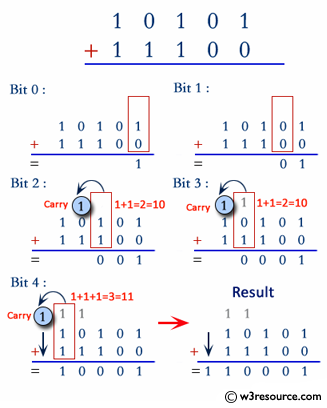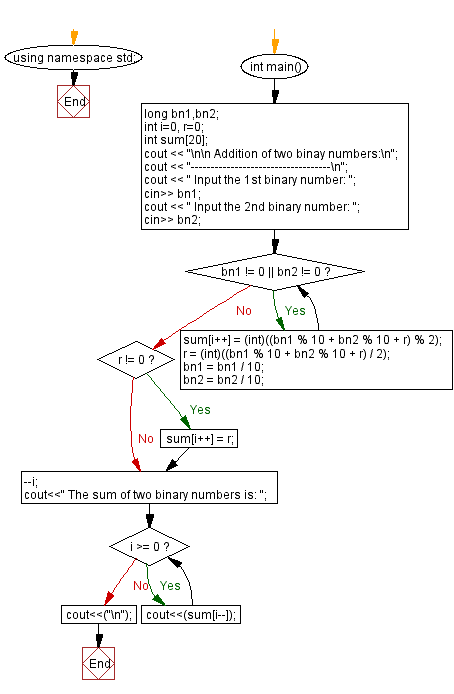﻿ C++ Exercises: Add two binary numbers - w3resource

# C++ Exercises: Add two binary numbers

## C++ Basic: Exercise-60 with Solution

Write a C++ program to add two binary numbers.

Pictorial Presentation:Sample Solution:

C++ Code :

``````#include <iostream>
#include <math.h>
using namespace std;

int main()
{
long bn1,bn2;
int i=0, r=0;
int sum;
cout << "\n\n Addition of two binary numbers:\n";
cout << "-----------------------------------\n";
cout << " Input the 1st binary number: ";
cin>> bn1;
cout << " Input the 2nd binary number: ";
cin>> bn2;
while (bn1 != 0 || bn2 != 0)
{
sum[i++] = (int)((bn1 % 10 + bn2 % 10 + r) % 2);
r = (int)((bn1 % 10 + bn2 % 10 + r) / 2);
bn1 = bn1 / 10;
bn2 = bn2 / 10;
}
if (r != 0) {
sum[i++] = r;
}
--i;
cout<<" The sum of two binary numbers is: ";
while (i >= 0) {
cout<<(sum[i--]);
}
cout<<("\n");
}
``````

Sample Output:

``` Addition of two binay numbers:
-----------------------------------
Input the 1st binary number: 1010
Input the 2nd binary number: 0011
The sum of two binary numbers is: 1101
```

Flowchart:C++ Code Editor:

What is the difficulty level of this exercise?

﻿

## C++ Programming: Tips of the Day

Why is there no std::stou?

The most pat answer would be that the C library has no corresponding "strtou", and the C++11 string functions are all just thinly veiled wrappers around the C library functions: The std::sto* functions mirror strto*, and the std::to_string functions use sprintf.

Ref: https://bit.ly/3wtz2qA

We are closing our Disqus commenting system for some maintenanace issues. You may write to us at reach[at]yahoo[dot]com or visit us at Facebook